Pandas GroupBy – Count occurrences in column

• Last Updated : 02 Jun, 2021

Using the size() or count() method with pandas.DataFrame.groupby() will generate the count of a number of occurrences of data present in a particular column of the dataframe. However, this operation can also be performed using pandas.Series.value_counts() and, pandas.Index.value_counts().

Approach

• Import module
• Create or import data frame
• Apply groupby
• Use any of the two methods
• Display result

Method 1: Using pandas.groupyby().size()

The basic approach to use this method is to assign the column names as parameters in the groupby() method and then using the size() with it. Below are various examples that depict how to count occurrences in a column for different datasets.

Example 1:

In this example, we separately count occurrences of all the columns present in a dataset.

Python3

 # import moduleimport pandas as pd  # assign datadata = pd.DataFrame({'Section': ['A', 'A', 'A', 'B', 'B',                                 'B', 'C', 'C', 'C'],                     'Teacher': ['Kakeshi', 'Kakeshi', 'Iruka',                                 'Kakeshi', 'Kakeshi', 'Kakeshi',                                 'Iruka', 'Iruka', 'Guy']}) # display dataframeprint('Data:')display(data)  print('Occurrence counts of particular columns:') # count occurrences a particular columnoccur = data.groupby(['Section']).size() # display occurrences of a particular columndisplay(occur) # count occurrences a particular columnoccur = data.groupby(['Teacher']).size() # display occurrences of a particular columndisplay(occur)

Output: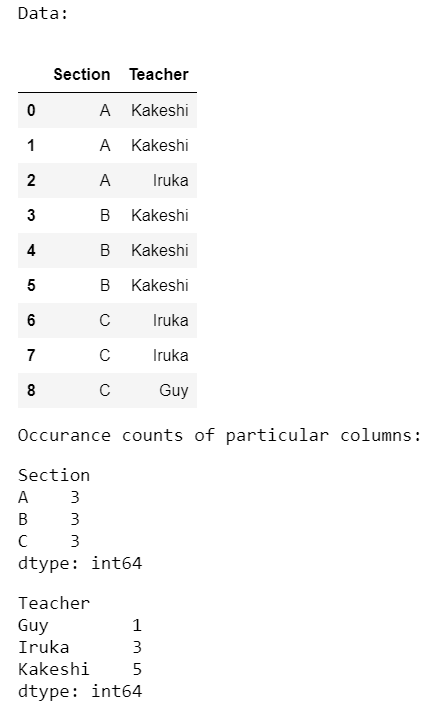Example 2:

In the below program, we count occurrences of all the columns combined from the same dataset as used in the previous program.

Python3

 # import moduleimport pandas as pd  # assign datadata = pd.DataFrame({'Section': ['A', 'A', 'A', 'B', 'B', 'B',                                 'C', 'C', 'C'],                     'Teacher': ['Kakeshi', 'Kakeshi', 'Iruka',                                 'Kakeshi', 'Kakeshi', 'Kakeshi',                                 'Iruka', 'Iruka', 'Guy']}) # display dataframeprint('Data:')display(data)  print('Occurrence counts of combined columns:') # count occurrences of combined columnsoccur = data.groupby(['Section', 'Teacher']).size() # display occurrences of combined columnsdisplay(occur)

Output:Example 3:

Here, we separate count occurrences and combined count occurrences of the categorical columns present in a CSV file.

Python3

 # import moduleimport pandas as pd  # assign datadata = pd.read_csv('diamonds.csv') # display dataframeprint('Data:')display(data.sample(10)) print('Occurrence counts of particular column:') # count occurrences a particular columnoccur = data.groupby(['cut']).size() # display occurrences of a particular columndisplay(occur) print('Occurrence counts of combined columns:') # count occurrences of combined columnsoccur = data.groupby(['clarity', 'color', 'cut']).size() # display occurrences of combined columnsdisplay(occur)

Output: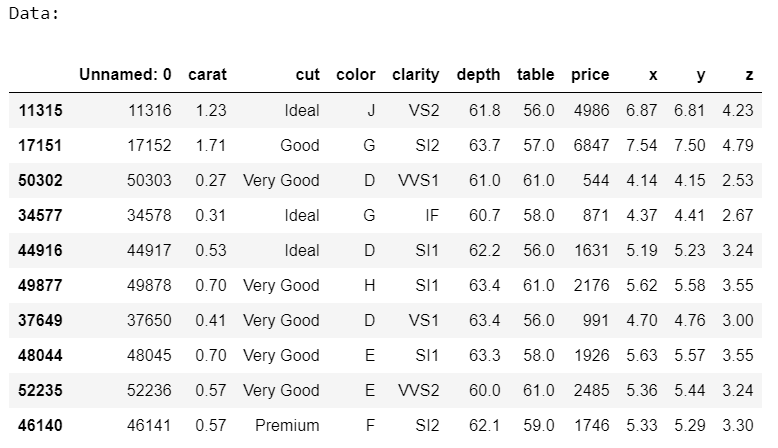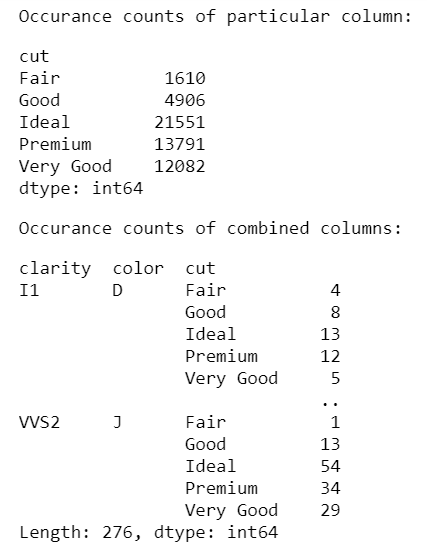Method 2: Using pandas.groupyby().count()

The basic approach to use this method is to assign the column names as parameters in the groupby() method and then using the count() with it. Below are various examples that depict how to count occurrences in a column for different datasets.

Example 1:

In this example, we separately count occurrences of all the columns present in a dataset.

Python3

 # import moduleimport pandas as pd  # assign datadata = pd.DataFrame({'Section': ['A', 'A', 'A', 'B', 'B', 'B',                                 'C', 'C', 'C'],                     'Teacher': ['Kakeshi', 'Kakeshi', 'Iruka',                                 'Kakeshi', 'Kakeshi', 'Kakeshi',                                 'Iruka', 'Iruka', 'Guy']}) # display dataframeprint('Data:')display(data) print('Occurrence counts of particular columns:') # count occurrences a particular columnoccur = data.groupby(['Section']).size() # display occurrences of a particular columndisplay(occur) # count occurrences a particular columnoccur = data.groupby(['Teacher']).size() # display occurrences of a particular columndisplay(occur)

Output: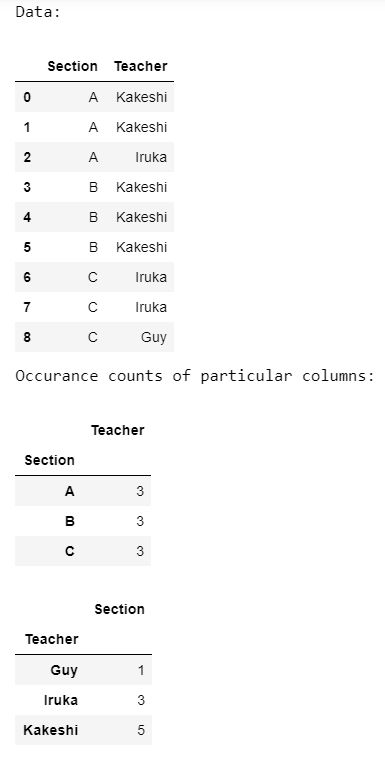Example 2:

In the below program, we count occurrences of all the columns combined from the same dataset as used in the previous program.

Python3

 # import moduleimport pandas as pd  # assign datadata = pd.DataFrame({'Section': ['A', 'A', 'A', 'B', 'B', 'B',                                 'C', 'C', 'C'],                     'Teacher': ['Kakeshi', 'Kakeshi', 'Iruka',                                 'Kakeshi', 'Kakeshi', 'Kakeshi',                                 'Iruka', 'Iruka', 'Guy']}) # display dataframeprint('Data:')display(data) print('Occurrence counts of combined columns:') # count occurrences of combined columnsoccur = data.groupby(['Section', 'Teacher']).size() # display occurrences of combined columnsdisplay(occur)

Output:Example 3:

Here, we separate count occurrences and combined count occurrences of the categorical columns present in a CSV file.

Python3

 # import moduleimport pandas as pd  # assign datadata = pd.read_csv('diamonds.csv') # display dataframeprint('Data:')display(data.sample(10))  print('Occurrence counts of particular column:') # count occurrences a particular columnoccur = data.groupby(['cut']).size() # display occurrences of a particular columndisplay(occur)  print('Occurrence counts of combined columns:') # count occurrences of combined columnsoccur = data.groupby(['clarity', 'color', 'cut']).size() # display occurrences of combined columnsdisplay(occur)

Output: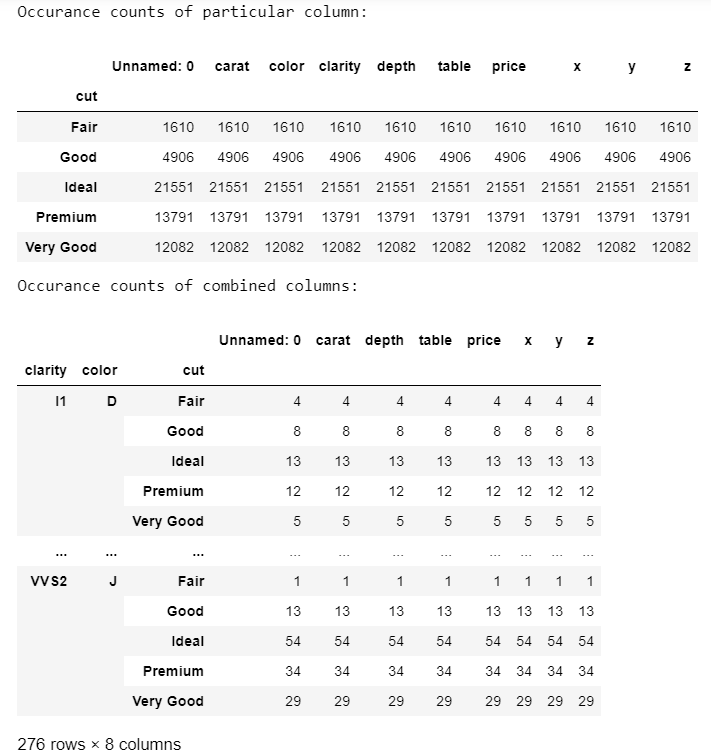My Personal Notes arrow_drop_up